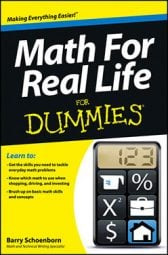##### Math For Real Life For DummiesWhen you go shopping, you're often faced with discounts and sales offers that change the price of an item. In addition, many states in the United States charge sales tax, and if you're shopping abroad, you may face a value added tax (VAT). To determin how much the total will be (and whether it fits into your budget or whether you have enough cash on hand to make the purchase), you need to be able to calculate the discounted price as well as determine the sales and any other taxes.

• Estimating sales tax: In the U.S., state sales taxes often end up being over 8 percent when you add in applicable local taxes. Therefore, a safe estimate is to allow for a 10 percent sales tax. A quick way to figure 10 percent of an item's purchase price is to move the decimal point one place to the left. For example, if you buy a \$70.00 item at the hardware store, the tax will be approximately \$7.00, making the purchase price in the neighborhood of \$77.00.

• Estimating VAT: Many European Union and non-EU countries have a value added tax (VAT) that hovers around 20 percent. To estimate VAT, follow the same approach as you would to calculate a sales tax: determine 10 percent by moving the decimal one place to the left. Then double that amount. Using the \$70.00 purchase at the hardware store example, the VAT will be approximately \$14.00.

• Calculating a discount: Discounts can be for any amount: 5 percent, 10 percent, 30 percent, and so on. To do the calculations in your head, first determine what a 10 percent discount is (because that's the easiest one to calculate) and then do a little multiplication or division to get closer to the actual discount amount.

For example, if an item is being offered at a 40 percent discount, figure 10 percent and then multiply that amount by 4. A 40 percent discount on a \$80.00 dress, for example, works out to be \$32.00 off (10 percent of \$80.00 is \$8.00, and \$8.00 multiplied by 4 is \$32.00). If the discount is 15 percent, figure the 10 percent discount, divide that number in half to determine what a 5 percent discount, and then add the two together. For the \$80.00 dress, you'd end up with a \$12.00 discount: \$8.00 (10 percent) + \$4.00 (5 percent) = \$12.00 (15 percent).